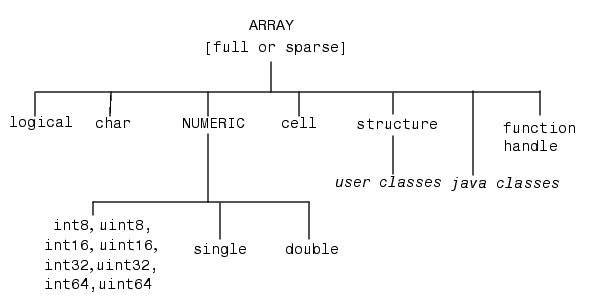## 15-1 嚙踝蕭嚙踝蕭嚙踝蕭嚙踝蕭嚙踝蕭嚙踝蕭嚙踝蕭嚙踝蕭嚙踝蕭嚙踝蕭嚙踝蕭嚙踝蕭

Hint

Example 1: 15-物件導向程式設計/@polynom/polynom.mfunction poly = polynom(vec) %POLYNOM Polynomial class constructor % poly = POLYNOM(vec) creates a polynomial object from the given vector vec % which contains the coefficients of the descending-order polynomial. if isa(vec, 'polynom') % 若 vec 已經是 polynom 物件，則直接設定成輸出 poly = vec; else poly.c = vec(:).'; % 將向量設定成 poly 的係數 poly = class(poly, 'polynom'); % 將 poly 加持成 polynom 物件 end

Example 2: 15-物件導向程式設計/@polynom/display.mfunction display(poly) % POLYNOM/DISPLAY Display of a polynom disp(' '); disp([inputname(1),' = ']) disp(' '); disp([' ', polyAsString(poly)]) disp(' ');

Example 3: 15-物件導向程式設計/@polynom/polyAsString.mfunction s = polyAsString(poly) % POLYNOM/POLYASSTRING String representation of a polynom degree=length(poly.c)-1; s = sprintf('%d*x^%d', poly.c(1), degree); for i=degree-1:-1:0 coef = poly.c(degree-i+1); if coef >=0 s=sprintf('%s + %d*x^%d', s, coef, i); else s=sprintf('%s - %d*x^%d', s, -coef, i); end end

Example 4: 15-物件導向程式設計/test01.mp = polynom([3 4 2 1]) p = 3*x^3 + 4*x^2 + 2*x^1 + 1*x^0

Hint